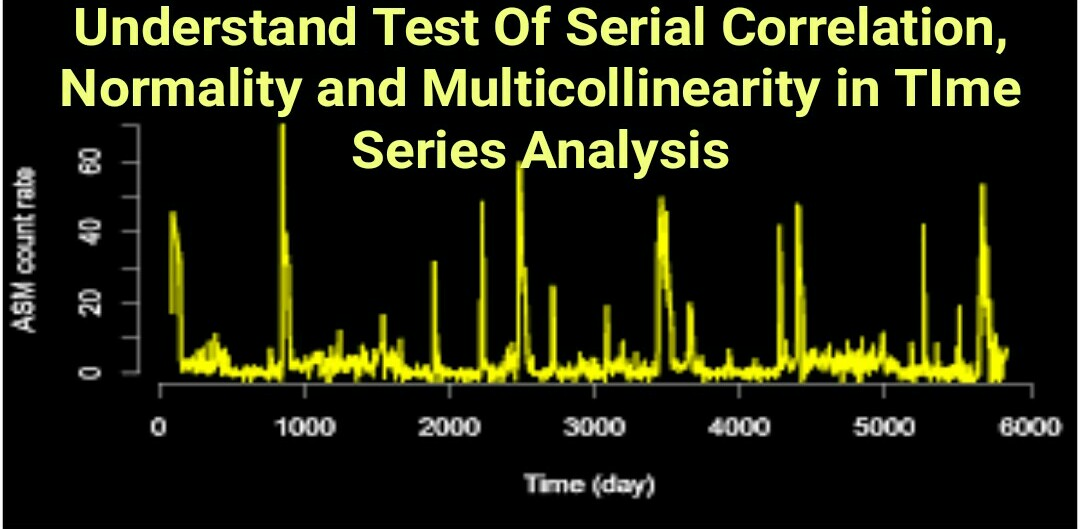--># Understand Test Of Serial Correlation, Normality and Multicollinearity in TIme Series Analysis

Understand Test Of Serial Correlation, Normality and Multicollinearity in TIme Series Analysis

Serial Correlation

Serial Correlation - correlation between ERROR TERM and INDEPENDENT variable. Tested with breusch-pagan regression (to see if change in independent variable significantly explains change in error term) or looking at the graph. Leads to std errors that are too low and t stats that are too high. Too many type 1 errors. Use robust standard to correct. If time serious, it is tested with ARCH but same principle applies, if ARCH model is significant, model is bad, use generalized least squares.

Serial Correlation

Serial Correlation - High correlation between previous errors and next errors. Tested by Durbin watson test. 2x(1-correlation) is an approximation. corr of +1 = DW of 0. corr of -1 = DW of 4 and u have negative serial corr. corr of 0 = DW of 2. So for short DW=0=positive serial corr. DW=2=no serial corr. DW=4=negative serial correlation. U have to look at critical values but most of the time its close enough to 0,2, or 4. Also has low std error, high t stats, high type 1 error. use robust std errors to correct. If its an AR model, look at autocorrelation in the lagged variables, if they're significant, add a lagged variable. For quarterly, it might be the 4th quarter, monthly data it might be the 12th.

Multicollinearity

Multicollinearity- Addition of an independent variable that is highly correlated with an existing independent variable. This inflates variance in the regression making std errors higher and t stats too low, more type 2 errors. Adding variables always increases R^2 so high R^2 and F-stat with insignificant t stats shows this. Is there are only 2 variables, the correlation between the two independent variables is the best indicator. If present, remove variable.

Dickey-Fuller Unit Root Tests

Dickey-Fuller Test

The Dickey-Fuller (DF) test was developed and popularized by Dickey and Fuller (1979). The null hypothesis of DF test is that there is a unit root in an AR model, which implies that the data series is not stationary. The alternative hypothesis is generally stationarity or trend stationarity but can be different depending on the version of the test is being used. Consider the following example to understand DF test.

Augmented Dickey–Fuller Test

The augmented Dickey–Fuller (ADF) test is a popular approach used for testing the unit root null hypothesis. The tests were performed on raw price indices and logarithm-transformed data in both levels and first differences. The ADF test employs the following regression model:

where Δ = the first difference operator; ΔYt−i = lagged values of the dependent variable, for example, ΔYt−1 = (Yt−1 − Yt−2), ΔYt−2 = (Yt−2 − Yt−3), and so forth; ɛt is a white noise error term; β1 is a constant; β2 is a slope coefficient on time trend t; δ is a coefficient of lagged Yt−1; and Yt is the logarithm of the stock price or market price index

The unit root tests described above are valid if the time series yt is well characterized by an AR(1) with white noise errors. Many financial time series, however, have a more complicated dynamic structure than is cap￾tured by a simple AR(1) model. Said and Dickey (1984) augment the basic autoregressive unit root test to accommodate general ARMA(p, q) models with unknown orders and their test is referred to as the augmented Dickey￾Fuller (ADF) test. The ADF test tests the null hypothesis that a time series yt is I(1) against the alternative that it is I(0), assuming that the dynamics in the data have an ARMA structure. The ADF test is based on estimating the test regression.

Phillips-Perron Unit Root Tests

Phillips and Perron (1988) developed a number of unit root tests that have become popular in the analysis of financial time series. The Phillips-Perron (PP) unit root tests differ from the ADF tests mainly in how they deal with serial correlation and heteroskedasticity in the errors. In particular, where the ADF tests use a parametric autoregression to approximate the ARMA structure of the errors in the test regression, the PP tests ignore any serial correlation in the test regression. The test regression for the PP tests is

∆yt = β0 Dt + πyt−1 + ut

where ut is I(0) and may be heteroskedastic. The PP tests correct for any serial correlation and heteroskedasticity in the errors ut of the test regression by directly modifying the test statistics tπ=0 and Tπˆ. These modified statistics, denoted Zt and Zπ, are given by